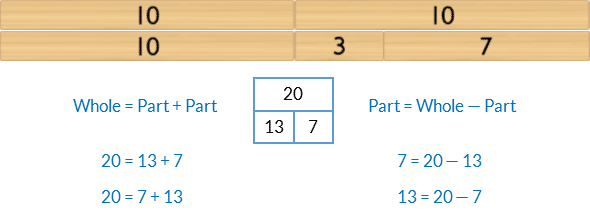## 46) Equation

Ten Plus Bonds: Building

### Mathematics

Develop the concept of:

• Two-part bonds of 20, where one part is a ten plus bond.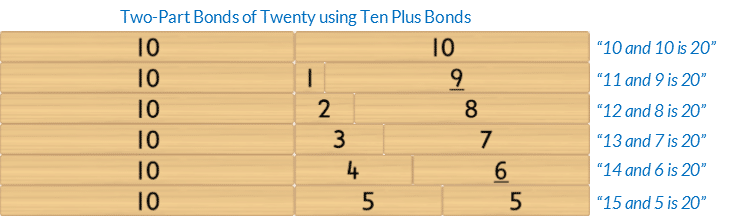• The associative property of addition: three or more parts can be added in any order without changing the size of the whole.
Each three-part bond of 20 is rearranged to make two, two-part bonds of 20.
Each two-part bond of 20 has one part that is a ten-plus bond.• Related addition and subtraction equations, rearranged using the part-part-whole relationship, for two-part bonds of 20, where one part is a ten plus bond.
• Mathematics as the science of pattern.

### Language

• “(Part) and (part) is (whole)” eg “19 and 1 is 20”
• three-part bond
• two-part bond
• ten plus bond
• bond of 10
• subtraction as “subtract”
• equals

### Core Activity Support MaterialsClick to open in a new tab.## Differentiation

### A little easier

When completing the core activity it is recommended that students complete the addition and subtraction equations working horizontally across the board one bond at a time. However, students with executive processing difficulties may find swapping between addition and subtraction confusing.

Adjust the activity by instructing students who have difficulty with this to complete the board vertically, filling the column of addition in one session, and the column of subtraction in a separate session.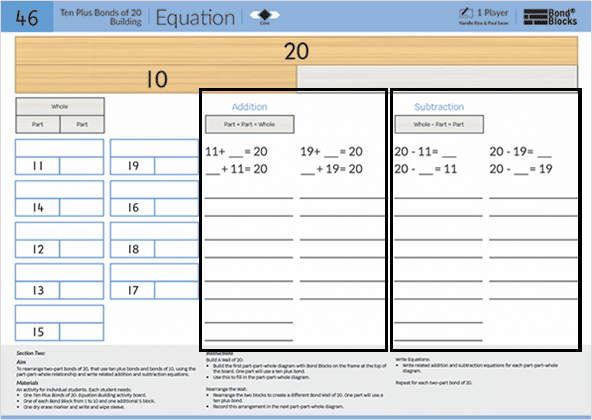##### Colour code related bonds of 10

After building the wall and verbalising the two-part bonds of 20 select four different coloured dry eraser markers.

• Circle the two-part bonds of 20 that use related bonds of 10.
• Verbalise these related bonds together. For example, “11 and 9 is 20 so 19 and 1 is 20”. Touch the related parts as they are said.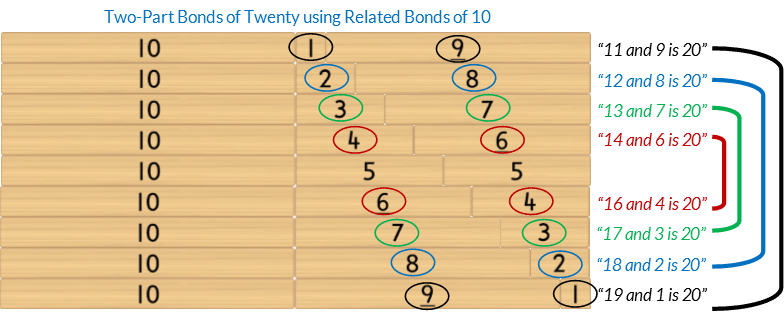### A little harder

##### Three-part bond equations.

Write equations using three part wholes of 20. One part in each equation must be a part of 10. Build the three-part whole using Bond Blocks then write as many addition and subtraction equations using those three parts as possible. For example,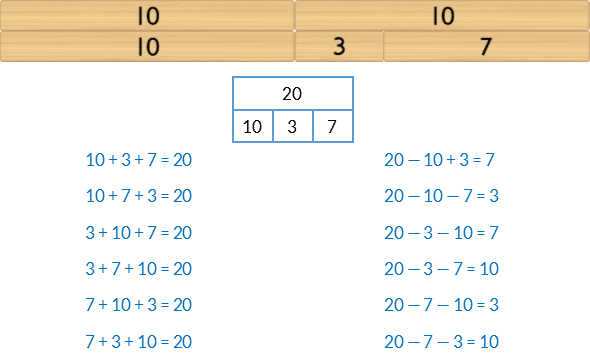##### Atypical arrangement

Addition and subtraction equations don’t have to be written as

Part + Part = Whole

Whole – Part = Part

An alternative arrangement is

Whole = Part + Part

Part = Whole – Part